# A Multi-class SVM Based Content Based Image Retrieval System Using Hybrid Optimization Techniques

A Multi-class SVM Based Content Based Image Retrieval System Using Hybrid Optimization Techniques
Department of ECE, Aditya College of Engineering and Technology, Surampalem, Andhra Pradesh 533437, India

Department of ECE, University College of Engineering Vizianagaram, JNTUK, Vizianagaram 535003, India

Corresponding Author Email:
kishore_dannina@acet.ac.in
Page:
217-226
|
DOI:
https://doi.org/10.18280/ts.370207
23 October 2019
|
Accepted:
5 February 2020
|
Published:
30 April 2020
| Citation

OPEN ACCESS

Abstract:

Due to the increasing usage of multimedia and storage devices accessible, searching for large image databases has become imperative. Furthermore, the handiness of high-speed internet has escalated the exchange of images by users enormously. Content-Based Image Retrieval is proposed in this work, taking features based on Exact Legendre Moments, HVS color quantization with dc coefficient and statistical properties such as variance, mean, and skew of Conjugate Symmetric Sequency Complex Hadamard Transform (CS-SCHT). In most of the machine learning tasks, the quality of the learning process depends on dimensionality. High dimensional datasets can influence the classification outcome and training time. To overcome this problem, we use DE (Differential Evolution) to generate the optimal feature subsets. The features scaled by weights derived from the firefly algorithm, which fed to Multi-Class SVM. The fitness function taken for the firefly algorithm is the classification error of SVM. By minimizing fitness function, optimum weights are obtained. When these optimal weights are applied to SVM, the proposed algorithm exhibits better precision, recall, and accuracy when compared to some of the existing algorithms in the literature.

Keywords:

CBIT, CS-SCHT, exact Legendre moments, HSV color quantization, differential evolution, multi-class SVM, firefly algorithm

1. Introduction

Nowadays, image archival has been tremendously increasing due to the diffusion of the internet, digitization of multimedia, and the freely available editing tools. In Google Images, the search made for finding similar images related to the Query image. Therefore, querying and indexing of the large database is increasingly becomes the prime concern in remote sensing, crime detection, publishing, medicine, architecture, multimedia, and education, etc. This concern is resolved by content-based image retrieval.

The content of an image may be either one of the three types viz., spatial, semantic, or low-level features as shown in Figure 1. Spatial content is characterized by the position of objects in the image, meaning represents semantic content and the Low-level features in the image are represented by texture or shape and color. A Content-Based Image Retrieval (CBIR) technique that relied on low-level features for efficient searching and retrieval is proposed in this paper. The most prominently used database for CBIR systems is Corel database. For a fair comparison, we used the same database, which consists of ten different categories of images. The performance evaluation metrics of an image retrieval system depends on feature selection, feature dimensionality reduction and the classifier employed. Shape information is more suitable for classification when compared to texture and color. However, the color content of an image plays a significant role in CBIR. The global histogram is famous for color content representation. Feature selections obtained by converting three (RGB) color channels into the HIS color standard.

Recently, CBIR is making use of convolutional neural networks (CNN’s) for improving retrieval performance. The features extracted from different layers of deep CNN are better compared to handcrafted features as the network of layers tries to minimize the error between actual and obtained results by adjusting the weights. However, the performance of retrieval in terms of training time and memory space is poor since these methods employ large sizes of vocabulary to build the image vectors. The time and memory complexity of CNN are very high since CNN models need to be pre-trained on thousands and millions of images.

All of these challenges motivated us to extract hand crafted low level features such as color, texture and shape from the image since the efficacy of the image retrieval system mainly depends on the features extracted from images. But all the extracted features are not necessary and only discriminative features that are useful for distinguishing one class of objects from the other required in classification as the irrelevant features can cause a low accuracy and increasing the computational time. Images in the Corel database contain different numbers of objects, background, illumination, and scenes. Such complex information of pictures represented by the multimodal representation problem cannot be solved by non-optimization algorithms. Hence, in this work, we used differential evolution for selecting the discriminative features. For the first time, the error in the SVM network controlled by random initial weights multiplied by a feature vector, and the cost function is minimized by reducing the error. There by the accuracy of the proposed algorithm is improved with the usage of optimization in discriminative feature selection and classification accuracy.

## 1.pngFigure 1. Visual content of image

Content-based image retrieval (CBIR) facilitates the efficient recovery of images related to the query model from the extensive image database. The features in this work are collected using transform coefficients of CS-SCHT. In the transform domain, the image represented in a compact form. The statistical features viz., mean, variance, and skew of CS-SCHT transform are computed. Later, these features are fused with the shape features of Exact Legendre Moments and color features based on HSV color quantization. In this work, the fused attributes are classified using Support Vector Machine. The classification error in SVM is reduced by adjusting the weights of the feature matrix by using the firefly algorithm. The efficacy of CBIR depends on features to search for images from massive image databases according to user requests in the form of a query image.

The rest of the paper is framed as follows. In section 2, a review of several content based image retrieval systems are presented. Proposed CBIR algorithm is discussed in section 3. Experimental results are presented in section 4 and it is followed by Section 5 that concludes the proposed method.

2. Related Work

Subramanyam et al.  proposed a CBIR using features of the Modified Color Motif co-occurrence matrix (MCMCM). The novelty of the work is that they considered intercorrelation between R, G, and B planes. Features from MCMCM and the difference between the pixels of a scan pattern (DBPSP) are combined in equal proportions to generate a feature vector. The computational complexity is very small, but getting of good performance is very difficult using MCMCM and DBPSP features. Daisy et al.  proposed CBIR by collecting texture and shape data calculated from the database images, and the query image and similarity index is measured using Euclidean distance. The feature vector made with morphological operations using a spatially variant structuring element. Though the performance of this method is good, higher computational complexity of Gabor wavelets and Fourier descriptor are the major limitations of this method. Lee et al.  proposed CBIR based on a weighted combination of color and texture to the Discrete Wavelet Transform (DWT). The advantage of this method is the average retrieval time is small. However, the lack of shape information in forming the feature vector is the major limitation of this approach. Wang et al.  proposed a method for CBIR by combining shape and texture. Shape features extracted for RGB color space using exponent moments and texture features obtained for HSI color space using localized angular phase histogram. The efficacy of this method is good but it depends on selection of moment order, which is manually done.

Feature reduction techniques also play an essential role in increasing the accuracy of the classifier. Various meta-heuristic search methods like simulated annealing, ant colony optimization, genetic algorithm, particle swarm optimization, and differential evolution for feature selection are available in the literature . Frohlich et al.  proposed a method in which the GA is used for feature selection and to optimize the regularization parameter of the SVM in parallel. This method is computationally attractive for determining the kernel parameters and an appropriate feature subset compared to Recursive Feature Elimination algorithm. The theoretical bounds on the generalization error for SVM is the major limitation of this method. The DE optimization technique as an advanced version of the real-valued GA that employs differential mutation operators with faster convergence properties. He et al.  suggest a discrete feature selection method using the DE algorithm, which uses a sigmoid limiting function for converting the real values into binary values, 0 and 1, that are obtained by using DE operators. After selection process experiments performed with SVM. Martinoyić et al.  proposed a method by combining Differential Evolution with k-Nearest Neighbor for pattern classification. In this, DE finds feature subsets from the complete set of features that were analyzed by the k-NN algorithm at that time, according to the method presented by Das et al. , and scale factor F is varied randomly.

Li et al.  proposed the CBIR system in which feature selection and parameter optimization obtained concurrently using SVM optimization. Hoi et al.  developed a CBIR system with a semi-supervised SVM batch mode active learning scheme. This scheme is to solve Relevant Feedback (RF) in CBIR. The performance of the SVM has improved irrespective of size and count of the labeled examples but it is applicable exclusively for CBIR with relevance feedback mechanism. Wang et al.  formulated an active-learning SVM-based RF using multiple classifier ensembles and features re-weighting. Zhang and Jiang et al.  proposed a method in which color and texture features used for image retrieval. According to the image texture characteristics, information of texture is represented by the fusion algorithm in combination with Gabor transform and edge histogram. The blocking and fuzzy color histogram proposed and CH in HSV color space chosen as a color feature. It is able to perform well and offers rotation and scale invariance. The representation is not compact because Gabor functions do not form an orthogonal basis set. Further, lack of shape information in forming the feature vector is another limitation of this method. Gupta et al.  proposed a method to combines global and local features. GLCM used for feature extraction, SVM for classification while Haar DWT utilized for partitioning an image into the horizontal, vertical, and diagonal regions. The efficiency of this system is improved based on low level features. However, the size of the feature vector is large. Further, the computational time is quite high. Yan et al.  introduced a novelty in the CBIR system by incorporating two strategies. The first strategy is samples chosen based on the similarity feature for active learning. The second strategy they employed is for parameter tuning using adaptive regularization that selects the optimal model. Active learning SVM combines the model selection with active-learning to obtain optimal classifier. Ashraf et al.  proposed a method for image retrieval using artificial neural networks in which features extracted by using bandlet transform. To evaluate the retrieval efficiency of the system, the authors used precision and recall. The most important capability of the proposed method is its attribute for identifying the most prominent objects in an image for the generation of feature vector. The computational complexity of this method is high compared to conventional CBIR techniques. Alsmadi  proposed a method for CBIR in which the features extracted from the image were color signature, structure, and texture color. Meantime, image retrieval based on the GA with iterated local search (ILS) similarity measure and simulations carried on the Corel database. The addition of the ILS algorithm with the GA has increased the exploitation process resulting an increase in fitness number, is the advantage of this method. Computation time for fitness function is quite high is the limitation of this method.

Mohamed et al.  introduced an image retrieval system based on Convolutional Neural Networks (CNN) and Support Vector Machine (SVM). In this work, they used convolutional neural network as an extractor of image features and generate convolution maps. Later, these maps are flattened and concatenated into a CNN code. These codes are used as a new feature vector for SVM training. When the SVM classiﬁer has been well trained, it performs the recognition step and takes new decisions on testing images with such automatically extracted features. Maria and Tefas  have proposed image retrieval system based on deep convolutional neural networks. In this method they used a deep CNN model to obtain the feature representation by using max-pooling and subsequently they retrain the network in order to produce more efficient compact image descriptors, which improve both the memory requirements and the retrieval performance relying on the available information. In this work, they suggested three basic model retraining approaches: the full unsupervised, relevance information and relevance feedback based on no information available, labels of the training dataset are available and the feedback from users is available respectively. All these approaches are applicable to other CNN architectures. The proposed method outperforms other CNN based retrieval techniques.

3. Proposed Work

The proposed CBIR system architecture is presented in Figure 2 comprises of the following functional blocks:

3.1    Image database Corel—1K

3.2    Feature database

3.3    Feature extraction

3.4    Query image

3.5    Image matching & Indexing

3.6    Retrieved images

3.1 Image database

This block contains images of the chosen database. Similarity distance measurement of query image with database images can be made pixel by pixel basis, which is computationally complex, and it may limit the performance of the CBIR system. Therefore, instead of taking pixel by pixel comparison, a compact representation of the image features is used. In the proposed work, the experiments are carried on Corel-1k dataset which contains 10 categories of images such as Building, Elephants, Mountains, African tribe people, Food, Beach, Horses, Buses, Flowers and Dinosaurs. Each category having 100 pictures and the size of each picture is 384 X 256 or 256 X 384 and a total of 1000 images available in it.

## 2.png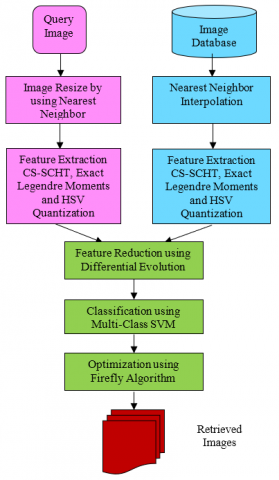Figure 2. Content based image retrieval using proposed algorithm

3.2 Feature database

Feature extraction plays a crucial role in the CBIR system. An image is characterized by a set of features, viz., texture, color, and shape. The feature consists of the hash extracted from a given image that can uniquely identify the image . The features are computed to the entire database images and stored in a feature database for matching and indexing.

3.3 Feature extraction

The first task in the CBIR system is feature extraction, and it is performed to extract visual features from an image to represent and index the image. A single attribute is not enough to discriminate among a homogeneous group of pictures so, a vector is used to represent an image that contains all its extracted features. The extracted features used in this work are statistical parameters of mean, standard deviation, and skew computed for dc values of CS-SCHT, shape features from Exact Legendre Moments, and HVS color quantization features. The computation of these features is shown below: As a preprocessing step, all the images in the database are resized to 256x256.

3.3.1 CBIR using the transform domain

The images in the Corel database are in RGB format. They are transformed into other color standards YCbCr and HSI. In this CS-SCHT is used to convert the pictures from spatial domain to frequency domain. This idea of using transformation is to reduce the redundancy so that most of the energy is packed in fewer coefficients . The size of the image in the transform and pixel domain is same and in transform domain, most of the coefficients are zero and significant information packed in the few non zero factors.

CS-SCHT is a complex orthogonal transform, and similar to Discrete Fourier Transform, the row vectors arranged in ascending order of sequency (number of sign changes in a row). The kernel coefficients of CS-SCHT are either one or j. It is a shift-invariant and conjugate symmetric spectrum. The computational complexity of CS-SCHT is low for real signals because the spectrum is conjugate symmetric, which in turn, half of the spectral coefficients are redundant. This transform applied to blocks of all the six planes of Y, Cb, Cr and H, S, I planes of images in database and query image. From each block, a dc value obtained, forming an array of transform coefficients. The mean, standard deviation, and skew computed for each color plane are shown in Algorithm 1.

 Algorithm 1: Feature Extraction using CS-SCHT Resize the image using nearest neighbor interpolation to size 256X256 Transform Color Space of an image from RGB to HIS and RGB to YCbCr. Apply CS-SCHT to non-overlapping blocks of size32 X 32 of each plane of Y, Cb, Cr and H, S, I. In each 32´32block, pick the DC coefficients. After finding the DC coefficients from each plane then find mean, standard deviation and skew. A total of 18 features are collected for each image.

3.3.2 Shape features using exact Legendre moments

Exact Legendre Moments (ELM's) are orthogonal, and these are used to represent the shape content of an image with compact representation. Orthogonal Moments have many desirable properties such as stability and fast numerical implementation due to recurrent relation in the computation of polynomials [21, 22]. The moments that are generated from these polynomials are more robust to noise. ELM of order 7 are shown in Figure 3.

## 3.jpg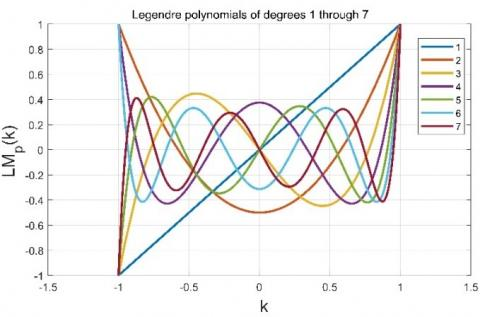Figure 3. The graphs of Legendre polynomials up to order 7

Orthogonal moments are invertible i.e., the original image can be reconstructed from moments. ELMs are rectangular polynomials and hence less computationally complex compared to the Zernike moments (ZMs). Exact Legendre moments of an image are given by

\begin{aligned} E L M_{p q}=\frac{(2 p+1)(2 q+1)}{4} & \int_{-1}^{1} \int_{-1}^{1} L M_{p}(k) L M_{q}(l) f(k, l) d k d l \\ p, q &=0,1,2 \end{aligned}    (1)

where $L M_{p}(k)$ is thepth degree Legendre Polynomial which can be represented by Rodrigues formula.

\begin{aligned} L M_{p}(k) &=\frac{1}{2^{p} p !} \frac{d^{p}}{d k^{p}}\left(k^{2-} 1\right)^{p} \\ & L M_{0}(k)=1 \\ & L M_{1}(k)=k \end{aligned}    (2)

$L M_{p+1}(k)=\frac{2 p+1}{p+1} k L M_{p}(k)-\frac{p}{p+1} L M_{p-1}(k)$    (3)

In this ELM of order 10 is used. So the feature size is 66.

3.3.3 Color features extraction using HSV color model

HSV color model is extensively used in color feature extraction. HSV color model perceives color in a way that is similar to human perception. It is evident that the color supplies robust descriptors for the CBIR method. Still, the Human Visual System (HVS) fails to recognize a large number of colors at the same time, but it is capable of differentiating similar shades accurately. The HSV color model has three components namely hue, saturation, and value. The hue component is a dominant attribute of color measured in angles. The amount of white light added to the dominant color termed as saturation. Pink is less saturated, and primary colors Red, Green, and blue are fully saturated colors. 'Value' is sometimes substituted with 'brightness'. According to the characteristics of HSV color space, the HVS parameters are quantized into 72 bins by dividing H into eight, S into three and V into another three regions as follows.

$H=\left\{\begin{array}{cc}\text { 0, } & \text { Hue } \in\left[0^{\circ}, 24^{\circ}\right] \cup\left[345^{\circ}, 360^{\circ}\right] \\ & \text { 1, } \text { Hue } \in\left[25^{\circ}, 49^{\circ}\right] \\ & \text { 2, Hue } \in\left[50^{\circ}, 79^{\circ}\right] \\ & \text { 3, Hue } \in\left\lceil 80^{\circ}, 159^{\circ}\text { ] } \right. \\ & \text { 4, } \text { Hue } \in\left\lceil 160^{\circ} \text { , } 194^{\circ}\text { ] } \right. \\ & \text { 5, Hue } \in\left\lceil 195^{\circ}, 264^{\circ}\text { ] } \right. \\ & \text { 6, Hue } \in\left[265^{\circ}, 284^{\circ}\right] \\ & \text { 7, Hue } \in\left[285^{\circ}, 344^{\circ}\right]\end{array}\right.$    (4)

$S=\left\{\begin{array}{ll}0, \text { Sat } \in & {[0,0.15]} \\ 1, \text { Sat } \in & {[0.15,0.8]} \\ 2, \text { Sat } \epsilon & {[0.8,1]}\end{array}\right.$   (5)

$V=\left\{\begin{array}{llrl}0, & V a l \in & {[0,0.15]} \\ 1, & V a l \in & {[0.15,0.8]} \\ 2, & V a l \in & {[0.8,1]}\end{array}\right.$    (6)

From the quantized values, an array of size 72 is formed as a feature vector. Let us assume that, $Q_{H}, Q_{S}$ and $Q_{v}$ are the quantized bins of H, S and V respectively. It is formed by

$P=Q_{s} Q_{v} H+Q_{v} S+V$    (7)

$Q_{s}$ and $Q_{v}$ are quantized into three levels. So, P can be obtained from Eq. (7) is

P=9 H+3 S+V   (8)

The above formula is simple and easy to determine similarity, which needs neither square nor square root operations. This formula can be used in our proposed method to determine similarity effectively.

3.3.4 Fusion of feature vector algorithm

 Algorithm 2: Generation of feature vector Mean, standard deviation and skew are computed from CS-SCHT transform dc coefficients. The number of features is 18. Shape features are extracted using Exact Legendre Moments with order 10, The number of features are 66 Color feature are extracted using HSV color model. The HVS parameters are quantized into 72 bins, by dividing H into 8 regions, S into 3 regions and V into 3 regions. The number of features is 72. Therefore, size of the feature vector is 18+66+72 = 156

3.4 Feature reduction

All the extracted features are not important for representing images and may be irrelevant and redundant. Therefore, to represent the image in a compact form, dimensionality reduction (DR) methods are used and these methods will choose the significant subset of features.

3.4.1 Feature reduction using DE

In recent research, DE is one of the best optimization techniques which has been popular, got attention among metaheuristic algorithms . It is the easiest optimizer based on population manipulating all parameters as floating point numbers with arithmetic operaters. The flowchart of DE based discriminant features selection from the collected feature set is shown in Figure 4.

The fore most step in DE is to produce a M-dimensional#real-valued parameter vector for each NP member in the population i.e., a matrix of dimension NP by M . A new mutant element is created by, the differential combination operator of element ‘r’ of vector ‘xq’ where, ‘q’ is population index which is in the range of 1 and Np, is obtained by summing the weighted difference between first element of two randomly selected population members xr,m  and xr,n  to the value of a third random member of the population xr,p. To enhance exploration, the dimension of the individual $v_{r, q}$ is changed with the corresponding dimension of xr,q with uniform probability CO.

$v_{r, q}=x_{r, p}+F \times\left(x_{r, m}-x_{r, n}\right)$    (9)

$x_{r, q}^{n e w}=\left\{\begin{array}{cc}v_{r, q} & \text { if rand }(0,1) \leq C O \\ x_{r, q} & \text { otherwise }\end{array}\right.$   (10)

where, factor F is a constant which controls the rate of the evolution of population, rand() is a uniformly distributed random number from [0,1] and CO is the crossover probability. Parameters are indexed with r, which ranges between 1 and D inside the vector. An adaptive scheme with good convergence is achieved by generating random deviations that are extracted from the population with both distance and direction information.

## 4.png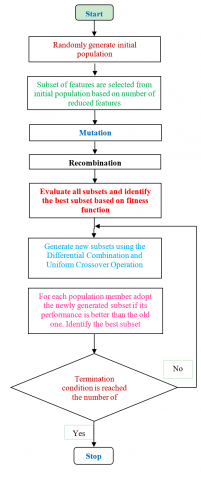Figure 4. Feature reduction using differential evolution

The fitness function used for DE is based on k-nearest neighbors. If a set X of some n points and a distance function are given, K-nearest neighbor search that find k closest points in X to a query point or set of points of Y. The fitness function must be supplied with the derived feature vectors from all the images, size of compact or reduced feature set, number of neighbors as the input and the output of fitness function is resubstitution loss. The DE strives for minimizing this loss until there is no change in the objective function or maximum numbers of generations are reached.

3.5 Classification

Usage of classifier eliminates the need for similarity measure in CBIR system. Images having similar feature are classified into one group by subjecting feature to the trained classifier. The most popular classifiers used in CBIR application are support vector machine (SVM), Bayesian classifier, neural network classifier and random forest.

SVM is a representative classifier, i.e., it used to solve the problems due to machine learning (ML). SVM supervised machine learning algorithm, which maximizes the space (margin) between hyperplane and data to minimize the upper bound of the generalization error. There are different ways to solve multi-class classification problems for SVM, such as DAG (Directed Acyclic Graph), BT (Binary Tree), OAO (One-Against One), and OAA (One Against All) classifiers. In the proposed method OAA strategy is used instead of OAO as OAA need less training models and gives comparable classification accuracy to OAO SVM [25-27]. Construction of one SVM per class is done by Multi-Class SVM, which helps to differentiate one class from other classes. Classification of an unknown pattern according to the maximum output among all SVMs. In this work, classification accuracy improved by minimizing classification error defining fitness function to the firefly algorithm.

During the past decade, nature inspired metaheuristic algorithms such as Firefly Algorithm (FA), Genetic Algorithm (GA), Particle Swarm Optimization (PSO) and Ant Colony Optimization (ACO) are mainly used algorithms for optimization . These algorithms have been very successful because of flexibility, simplicity, local optima avoidance and derivation free mechanism. Among various metaheuristics algorithms, FA is a bio-inspired behavior of firefly insect. They communicate with each other by emitting lights from their bodies and changing intensity accordingly. The efficacy of FA depends two major parameters such as light intensity and attractiveness between fireflies . Flashing light intensity changes from each resource according to the brightness of the firefly, which is represented and calculated with a kind of fitness function. Attractiveness of each firefly is calculated using Eq. (11) .

$\alpha(r)=\alpha_{0} e^{-\gamma d 2}$    (11)

where, $\alpha_{0}$ is the attractiveness at distance (d) = 0, γ is the absorption coefficient of light in air and d is the distance which is used to measure how two fireflies are attracted to each other and is calculated by Cartesian distancesmethodaas shown in Eq. (12).

$d_{i j}=\left\|p_{i}-p_{j}\right\|=\sqrt{\sum_{k=1}^{n}\left(p_{i}-p_{j}\right)^{2}}$    (12)

where, n indicates the dimensionality of the problem. Once a calculating the distances between the two fireflies, suppose the firefly sat position is having less flashing light intensity than firefly at position j then attractiveness occurs among them while moving the firefly position towards the firefly at position j. The following equation  reins this type of movement and it is represented as

$p_{i+1}^{i t}=p_{i}^{i t}+\alpha_{o} e^{\left(\gamma d_{i j}^{2}\right)} *\left(p_{j}^{i t}-p_{i}^{i t}\right)+\beta *\left(\operatorname{rand}-\frac{1}{2}\right)$   (13)

where, it represents number of iterations and β represents random numbers controlling the size of the random walk and rand represents random number generator which falls between . The firefly with low light intensity moves towards the higher one after consider in gits position and random walk is determined by a random generator multiplied by β  as well as attraction factor $\alpha_{0}$. The flow chart of FA is given in Figure 5.

Fitness Function: In this algorithm, the number of features is reduced to 10 using differential evolution algorithm. The reduced features are multiplied with weighted matrix denoted by w1, w2 …., w10. Instead of features applied to SVM, the weighted feature vector is fed to SVM. The kernel used in SVM is Gaussian kernel. The error of SVM is minimized. So the fitness function is taken as Mean Square Error of SVM. The weights are derived from the FA. When the optimum weights are given, the algorithm obtains improved accuracy.

$L=\sum_{j=1}^{n} w_{j} I\left\{\widehat{y}_{J} \neq y_{j}\right\}$   (14)

## 5.png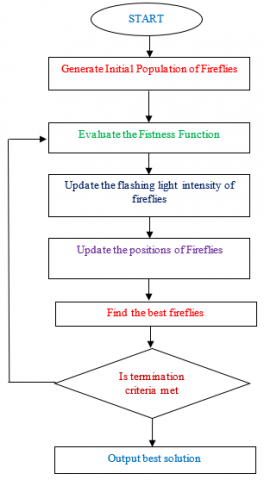Figure 5. Firefly algorithm flowchart

4. Results and Discussion

The proposed CBIR system in this analysis underwent tests with few images as queries. Then, similar images for a given query image extracted from the Corel-1k database. This algorithm uses features extracted through Exact Legendre Moments, HVS color quantization with dc coefficients statistical properties such as mean, variance, and skew of Conjugate Symmetric Sequency Hadamard Transform (CS-SCHT). The query image and the corresponding similar images retrieved from the database shown in Figure 6 and Figure 7.

## 6.jpg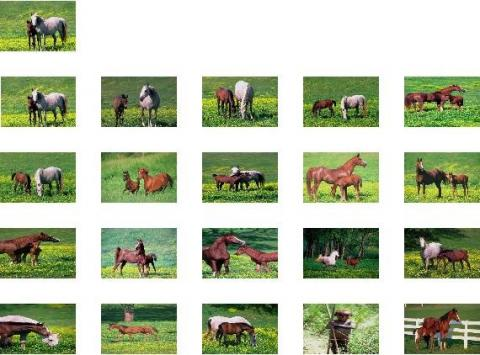Figure 6. Horse query image and retrieved horse images

## 7.jpg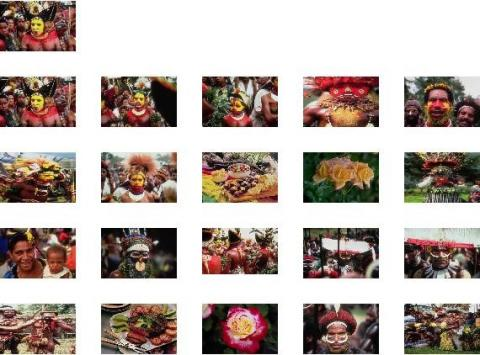Figure 7. African people query image and retrieved images

The color features calculated by using HVS color quantization and the features which represent shape were determined using the Exact Legendre moments. Apart from color and shape, we also determine statistical properties such as variance, mean, and skew of CS-SCHT. The extracted feature dimensions (156 features) were reduced by using Differential Evolution (DE) and generate a feature subset of size 10. After feature extraction subsets of dataset images, the comparison done with query image features. After the completion of the extraction of features, classification has done with unsupervised classifier i.e., MC-SVM. To validate the efficacy of our suggested image retrieval system, we took

“Corel 1k” as the image data set, and it contains 10 categories such as Building, Elephants, Mountains, African tribe people, Food, Beach, Horses, Buses, Flowers and Dinosaurs. Each category of Corel 1k consisting of 100 pictures, giving a total of 1,000 pictures in the dataset.

4.1 Retrieval evaluation

Specificity also called precision, which refers to the capacity measure of a system in image retrieving, which likely to query images. At the same time, sensitivity is also known as recall rate, gauges the potential of CBIR systems in the retrieval of the images that are identical to query images (QI’s). The computations on precision and recall measurements based on the Number of query images (from the test dataset) and the retrieval images from the Corel-1k database carried to elaborate results.

## 8.png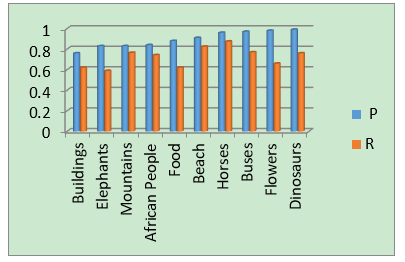Figure 8. Precision recall graph

The graphical representation of the P-R of different QI of the proposed CBIR system shown in Figure 8. As from the graph shown, the presented CBIR system has shown favorable results in terms of P-R. The experimental results through the extracted features which combined with the DE technique, Support Vector Machine, and Firefly algorithm shows improved accuracy of the CBIR method.

4.2 Evaluation on Corel image set

The performance of the proposed CBIR system measured with the Number of current content-based image retrieval systems [16, 17, 29-35]. The incentive of preferring this method is for comparison of results of all the methods reported via wang database of 10 categories such as Building, Mountains, African tribe people, Food, Beach, Horses, Buses, Flowers, and Dinosaurs. Each category of Corel-1k consisting of 100 pictures, giving a total of 1000 pictures in the dataset. The comparison of the average precision (AP). And average recall (AR) for the presented system with the other systems which compared can also be referable in Table 1 and Table 2.

As from the evidence, the above comparison results demonstrate the capacity of the proposed system in generating better precision and recall rates. Its performance also supersedes other state of the art works [16, 17, 29-35] with respect to precion and recall rates. In particular, the obtained AP, AR rates were 0.895 and 0.722. This is factored by the fact that the authors [16, 17, 29-35] developed CBIR systems based on restricted number of feature sets which restricts the retrieval efficacy. On the other hand, the proposed system in this work extracted robust and extensive set of features. In this method, color features using HVS color quantization, shape features using Exact Legendre Moments and texture features using CS-SCHT, are employed. The addition of Meta-heuristic algorithms; DE and FA for optimized discriminant features has improved retrieval efficiency by producing optimal weights. Clearly, the experimental results demonstrating the capacity of the   hybridization of two optimization algorithms in assisting the retrieval of the great amount of relevant images to the query image.

Table 1. AP results of the presented method compared with the current retrieval methods

 Category Proposed Method  Method  Method  Method  Method  Method  Method  Method  Method  Buildings 0.76 0.75 0.63 0.75 0.75 0.7 0.61 0.57 0.79 0.9 Elephants 0.83 0.83 0.72 0.9 0.8 0.7 0.57 0.67 0.71 0.7 Mountains 0.83 0.82 0.811 0.7 0.75 0.74 0.51 0.53 0.67 0.7 African People 0.84 0.83 0.82 0.8 0.65 0.64 0.56 0.7 0.81 0.9 Food 0.88 0.87 0.87 0.8 0.75 0.81 0.69 0.74 0.77 0.9 Beach 0.91 0.9 0.89 0.75 0.7 0.64 0.53 0.56 0.66 0.6 Horses 0.96 0.96 0.95 0.9 0.9 0.95 0.7 0.3 0.98 1 Buses 0.97 0.96 0.846 0.9 0.95 0.92 0.89 0.87 0.96 0.75 Flowers 0.98 0.96 0.91 0.8 0.95 0.95 0.89 0.91 0.97 0.9 Dinosaurs 0.99 0.99 0.82 0.97 1 0.99 0.98 0.91 1 1 Average 0.895 0.887 0.826 0.827 0.74 0.804 0.693 0.676 0.832 0.835

Table 2. The AR results of the presented method compared with the current retrieval methods

 Category Proposed Method  Method  Method  Method  Method  Method  Method  Method  Buildings 0.62 0.62 0.585 0.15 0.15 0.14 0.18 0.16 0.18 Elephants 0.589 0.58 0.533 0.18 0.16 0.16 0.15 0.14 0.14 Mountains 0.765 0.75 0.73 0.14 0.15 0.15 0.22 0.13 0.14 Africa 0.742 0.73 0.706 0.16 0.13 0.13 0.15 0.16 0.18 Food 0.62 0.62 0.6 0.16 0.15 0.16 0.13 0.15 0.18 Beach 0.825 0.815 0.805 0.15 0.14 0.13 0.19 0.13 0.12 Horses 0.875 0.85 0.848 0.18 0.1 0.19 0.13 0.13 0.21 Buses 0.77 0.75 0.73 0.18 0.19 0.18 0.11 0.19 0.17 Flowers 0.66 0.66 0.647 0.16 0.19 0.19 0.11 0.2 0.18 Dinosaurs 0.76 0.75 0.726 0.2 0.2 0.2 0.09 0.2 0.21 Average 0.722 0.712 0.691 0.166 0.164 0.163 0.146 0.159 0.171

Table 3. Average retrieval time for top 20 images in Corel-1k database

 Method Features Used Fitness Function Average Retrieval Time in Seconds  Color Histogram, Color Texture Features, Gray Level Cooccurrence Matrix (GLCM) and Canny Edge detector Squared Euclidean Distance 358.8  Color Histogram, Color Moments, Cooccurrence Matrix, Inverse Difference Moment, Wavelet Moments and  k-means clustering Evaluate a particle fitness based on clustering i.e. distance between query image and cluster center 381.62 Proposed HSV color histogram, Exact Legendra Moments and CS-SCHT features Mean Square error of SVM 249.56

4.3 Evaluation on average retrieval time

Feature extraction and average retrieval time is an important factor that directly influence the number of retrieved images. Table 3 shows the average retrieval time for top 20 images retrieved from the corel-1k dataset. The superiority of the proposed algorithm in terms of average retrieval time is observed over method  and method .

5. Conclusion

This paper proposes a hybrid framework for CBIR system using features calculated from CS-SCHT dc coefficients, Color features, and shape characteristics. The strength of the algorithm comes from robust feature selection using HVS color quantization, ELMs, and statistical parameters. Discriminant feature selection using DE and error minimization in SVM using a firefly algorithm with well-defined objective functions. The suggested system has experimented on the Corel-1k image database, and results showed that the recommended CBIR system performs well compared with the existing methods regarding average precision (AP) and the average recall (AR). The AP and AR values obtained with the suggested system were 0.895 and 0.722.

References

 Subrahmanyam, M., Wu, Q.J., Maheshwari, R.P., Balasubramanian, R. (2013). Modified color motif co-occurrence matrix for image indexing and retrieval. Computers & Electrical Engineering, 39(3): 762-774. https://doi.org/10.1016/j.compeleceng.2012.11.023

 Daisy, M.M.H., Selvi, S., Mol, J.G. (2013). Combined texture and shape features for content based image retrieval. In 2013 International Conference on Circuits, Power and Computing Technologies (ICCPCT), pp. 912-916. https://doi.org/10.1109/ICCPCT.2013.6528956

 Lee, Y.H., Rhee, S., Kim, B. (2012). Content-based image retrieval using wavelet spatial-color and Gabor normalized texture in multi-resolution database. Sixth International Conference on Innovative Mobile and Internet Services in Ubiquitous Computing, Palermo, pp. 371-377. https://doi.org/10.1109/IMIS.2012.98

 Wang, X.Y., Liang, L.L., Li, Y.W., Yang, H.Y. (2017). Image retrieval based on exponent moments descriptor and localized angular phase histogram. Multimedia Tools and Applications, 76(6): 7633-7659. https://doi.org/10.1007/s11042-016-3416-0

 Bonabeau, E., Dorigo, M., Marco, D.D.R.D.F., Theraulaz, G., Théraulaz, G. (1999). Swarm intelligence: From Natural to Artificial Systems (No. 1). Oxford University Press.

 Frohlich, H., Chapelle, O., Scholkopf, B. (2003). Feature selection for support vector machines by means of genetic algorithm in 15th IEEE International Conference on Tools with Artificial Intelligence, Sacramento, CA, USA, pp. 142-148. https://doi.org/10.1109/TAI.2003.1250182

 He, X., Zhang, Q., Sun, N., Dong, Y. (2009). Feature selection with discrete binary differential evolution. In 2009 International Conference on Artificial Intelligence and Computational Intelligence, Shanghai, pp. 327-330. https://doi.org/10.1109/AICI.2009.438

 Martinoyić, G., Draźen, B., Zorić, B. (2014). A differential evolution approach to dimensionality reduction for classification needs. International Journal of Applied Mathematics and Computer Science, 24(1): 111-122. https://doi.org/10.2478/amcs-2014-0009

 Das, S., Konar, A., Chakraborty, U.K. (2005). Two improved differential evolution schemes for faster global search. Proceedings of the 2005 Conference on Genetic and Evolutionary Computation, Washington DC, USA, pp. 991-998. https://doi.org/10.1145/1068009.1068177

 Li, J., Ding, L., Li, B. (2016). Differential evolution-based parameters optimisation and feature selection for support vector machine. Int. J. Computational Science and Engineering, 13(4): 355-363. https://doi.org/10.1504/IJCSE.2016.080212

 Hoi, S.C., Jin, R., Zhu, J., Lyu, M.R. (2009). Semisupervised SVM batch mode active learning with applications to image retrieval. ACM Transactions on Information Systems (TOIS), 27(3): 1-29. https://doi.org/10.1145/1508850.1508854

 Wang, X., Zhang, B., Yang, H. (2013). Active SVM-based relevance feedback using multiple classifier ensemble and features re-weighting. Engineering Applications of Artificial Intelligence, 26(1): 368-381. https://doi.org/10.1016/j.engappai.2012.05.008

 Zhang, H., Jiang, X. (2015). A method using texture and color feature for content-based image retrieval. In 2015 IEEE International Conference on Computer and Communications (ICCC), Chengdu, pp. 122-127. https://doi.org/10.1109/CompComm.2015.7387552

 Gupta, E., Kushwah, R.S. (2015). Combination of global and local features using DWT with SVM for CBIR. In 2015 4th International Conference on Reliability, Infocom Technologies and Optimization (ICRITO) (Trends and Future Directions), Noida, pp. 1-6. https://doi.org/10.1109/ICRITO.2015.7359320

 Yan, L., Xu, X.Y., Qi, G.H. (2015) Combining active learning and semi-supervised learning to construct SVM classifier. Knowledge-Based Systems, 44: 121-131. https://doi.org/10.1016/j.knosys.2013.01.032

 Ashraf, R., Bashir, K., Irtaza, A., Mahmood, M.T. (2015). Content based image retrieval using embedded neural networks with bandletized regions. Entropy, 17(6): 3552-3580. https://doi.org/10.3390/e17063552

 Alsmadi, M.K. (2018). Query-sensitive similarity measure for content-based image retrieval using meta-heuristic algorithm. Journal of King Saud University Computer and Information Sciences, 30(3): 373-381. https://doi.org/10.1016/j.jksuci.2017.05.002

 Mohamed, O., Khalid, E., Mohammed, O., Aksasse, B. (2017). Content-based image retrieval using convolutional neural networks. In: Mizera-Pietraszko J., Pichappan P., Mohamed L. (eds) Lecture Notes in Real-Time Intelligent Systems. RTIS 2017. Advances in Intelligent Systems and Computing, 756: 463-476. https://doi.org/10.1007/978-3-319-91337-7_41

 Maria, T., Tefas, A. (2018). Deep convolutional learning for content based image retrieval. Neurocomputing, 275: 2467-2478. https://doi.org/10.1016/j.neucom.2017.11.022

 Meenakshi, K., Swaraja, K., Kora, P. (2019). A robust DCT-SVD based video watermarking using zigzag scanning. Soft Computing and Signal Processing, 900: 477-485. https://doi.org/10.1007/978-981-13-3600-3_45

 Rao, C., Kumar, S.S., Mohan, B.C. (2010). Content based image retrieval using exact Legendre moments and support vector machine. arXiv preprint arXiv:1005.5437.

 Camacho-Bello, C. (2018). Exact Legendre–fourier moments in improved polar pixels configuration for image analysis. IET Image Processing, 13(1): 118-124. https://doi.org/10.1049/iet-ipr.2018.5489

 Price, K.V., Storn, R.M., Lampinen, J.A. (2005). Differential Evolution: A Practical Approach to Global Optimization. Springer.

 Storn, R. (2008). Differential evolution research – trends and open questions. In: Chakraborty U.K. (eds) Advances in Differential Evolution. Studies in Computational Intelligence, 143: 1-31. https://doi.org/10.1007/978-3-540-68830-3_1

 Ponmani, S. Samuel, Roxanna, Vidhu Priya, P. (2017). Classification, algorithms in data mining – A survey. Int. J. Adv. Res. Comput. Eng.Technol. (IJARCET).

 Usha, S.G.A., Vasuki, S. (2019). A novel method for segmentation and change detection of satellite images using proximal splitting algorithm and multiclass SVM. Journal of the Indian Society of Remote Sensing, 47(5): 853-865. https://doi.org/10.1007/s12524-019-00941-7

 Alam, J., Alam, S., Hossan, A. (2018). Multi-stage lung cancer detection and prediction using multi-class SVM classifier. International Conference on Computer, Communication, Chemical, Material and Electronic Engineering (IC4ME2), Rajshahi, pp. 1-4. https://doi.org/10.1109/IC4ME2.2018.8465593

 Wang, B., Li, D.X., Jiang, J.P., Liao, Y.H. (2016). A modified firefly algorithm based on light intensity difference. Journal of Combinatorial Optimization, 31(3): 1045-1060. https://doi.org/10.1007/s10878-014-9809-y

 Ashraf, R., Bashir, K., Mahmood, T. (2016). Content-based image retrieval by exploring bandletized regions through support vector machines. Journal of Information Science and Engineering, 32(2): 245-269.

 Youssef, S.M. (2012). ICTEDCT-CBIR: Integrating curvelet transform with enhanced dominant colors extraction and texture analysis for efficient content-based image retrieval. Computer & Electrical Engineering, 38(5): 1358-1376. https://doi.org/10.1016/j.compeleceng.2012.05.010

 Rao, M.B., Rao, B.P., Govardhan, A. (2011). CTDCIRS: content based image retrieval system based on dominant color and texture features. International Journal of Computer Applications, 18(6): 40-46. https://doi.org/10.5120/2285-2961

 ElAlami, M.E. (2011). A novel image retrieval model based on the most relevant features. Knowledge-Based Systems, 24(1): 23-32. https://doi.org/10.1016/j.knosys.2010.06.001

 Madhavi, K.V., Tamilkodi, R., Sudha, K.J. (2016). An innovative method for retrieving relevant images by getting the top-ranked images first using interactive genetic algorithm. Procedia Computer Science, 79: 254-261. https://doi.org/10.1016/j.procs.2016.03.033

 Pavithra, L.K., Sharmila, T.S. (2018). An efficient framework for image retrieval using color, texture and edge features. Computers & Electrical Engineering, 70: 580-593. https://doi.org/10.1016/j.compeleceng.2017.08.030

 Ahmed, K.T., Ummesafi, S., Iqbal, A. (2019). Content based image retrieval using image features information fusion. Information Fusion, 51: 76-99. https://doi.org/10.1016/j.inffus.2018.11.004

 Younus, Z.S., Mohamad, D., Saba, T., Alkawaz, M.H., Rehman, A., Al-Rodhaan, M., Al-Dhelaan, A. (2015). Content-based image retrieval using PSO and k-means clustering algorithm. Arabian Journal of Geosciences, 8(8): 6211-6224. https://doi.org/10.1007/s12517-014-1584-7